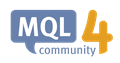# ChartXYToTimePrice50

Hello,

Bellow is the Sample EA code  found on https://docs.mql4.com/chart_operations/chartxytotimeprice.

Currently, Y axis numbers are descending as they follow the arrow upwards

Can you please amend the code so that Y axis numbers are ascending as they follow the arrow...upwards.

X axis is OK ,  Why? I'm importing XY values into  www.desmos.com/calculator  and their XY axes are counting from 0 upwards following the arrows.

Thank you very much !

luc

```//+------------------------------------------------------------------+
//| ChartEvent function                                              |
//+------------------------------------------------------------------+
void OnChartEvent(const int id,
const long &lparam,
const double &dparam,
const string &sparam)
{
//--- Show the event parameters on the chart
Comment(__FUNCTION__,": id=",id," lparam=",lparam," dparam=",dparam," sparam=",sparam);
//--- If this is an event of a mouse click on the chart
if(id==CHARTEVENT_CLICK)
{
//--- Prepare variables
int      x     =(int)lparam;
int      y     =(int)dparam;
datetime dt    =0;
double   price =0;
int      window=0;
//--- Convert the X and Y coordinates in terms of date/time
if(ChartXYToTimePrice(0,x,y,window,dt,price))
{
PrintFormat("Window=%d X=%d  Y=%d  =>  Time=%s  Price=%G",window,x,y,TimeToString(dt),price);
//--- Perform reverse conversion: (X,Y) => (Time,Price)
if(ChartTimePriceToXY(0,window,dt,price,x,y))
PrintFormat("Time=%s  Price=%G  =>  X=%d  Y=%d",TimeToString(dt),price,x,y);
else
Print("ChartTimePriceToXY return error code: ",GetLastError());
//--- delete lines
ObjectDelete(0,"V Line");
ObjectDelete(0,"H Line");
//--- create horizontal and vertical lines of the crosshair
ObjectCreate(0,"H Line",OBJ_HLINE,window,dt,price);
ObjectCreate(0,"V Line",OBJ_VLINE,window,dt,price);
ChartRedraw(0);
}
else
Print("ChartXYToTimePrice return error code: ",GetLastError());
Print("+--------------------------------------------------------------+");
}
}```ChartXYToTimePrice - Chart Operations - MQL4 Reference
• docs.mql4.com
//| ChartEvent function                                              |22709

lucian007: Currently, Y axis numbers are descending as they follow the arrow upwards

Of course, they do, top of the window is Y=0. Subtract from window height.50

Well, subtracting from window height is not an optimal option, takes extra time and is prone to miscalculation down the road.

Isn't it a better way, a code solution to make the Y scale starting from 0 same as X scale do ?

Your support is greatly appreciated !

lucModerator
24861

lucian007:

Well, subtracting from window height is not an optimal option, takes extra time and is prone to miscalculation down the road.

Isn't it a better way, a code solution to make the Y scale starting from 0 same as X scale do ?

Your support is greatly appreciated !

luc

Y does start from 050

...starts from 0 but from the screen upper left corner ?

Do you find it normal that Y scale point to its 0 (instead of infinite) as moves upwards and  X scale point to its infinite (rightly so)  as it moves to the right  ?

I didn't see such a weird chart model like this.

Indeed it prints some XY values of its own but I can't import them into a normal XY chart like desmos chart... Y scales point differently so obviously the result is different.

All is needed is that MT4 and desmos XY scales are a match in heaven ...common sense right ?

I hope that the issue is clear now.

Once again your help is highly appreciated !

lparam=x

dparam=y